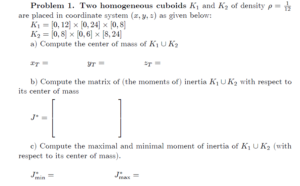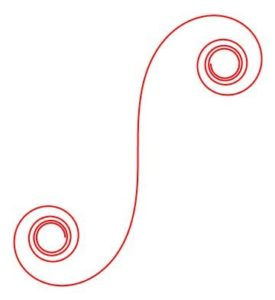# MATHEMATICS C (VECTOR CALCULUS)

GENERAL DESCRIPTION OF THE COURSE

The course consists of three main parts: vector functions and all about the curves (see figure on the right below) in 3D-space, partial derivatives (with applications like linearization, (constrained) optimization of functions of several variables) and integrals in (3D-space) space. Integrals in the space include not just the triple integral, but also surface and curve integrals with applications in physics. All calculations for the (individual) homework (see figure on the left below) are done with Scientific Notebook, which is on this level very useful in power computing.

In case that less than 5 Erasmus students are enrolled, the students are invited to attend the course in Slovene language (where the lecturer emphasizes the important things in English language) and have weekly meetings with the lecturer and assistant. All materials for Erasmus students: Exam, (Individual) Homework exercises, theoretical tests are in English language. Erasmus students have the same obligations as regular students. The students are also invited to attend the tutorials to be well-prepared for the written exam.

COURSE CONTENTS

The course will cover the following topics:

• VECTOR FUNCTIONS: curves in space, derivatives, connections with physics, natural parameter, curvatures.
• PARTIAL DERIATIVES AND APPLICATIONS: scalar fields, level curves, directional derivatives, gradient and maximal derivative, extremal problems, Hesse matrix, classification of extrema, extremal values on boundary, Lagrange multipliers, Taylor series in several variables, vector fields, Jacoby matrix, chain rule and applications, divergence, curl
• INTEGRALS IN SPACE AND APPLICATIONS: curve differential, length, work, potential, surface differential, area, flux, cylindrical and spherical coordinates, volume integrals, center of mass, moments, principal axes of curves, surfaces and solids, Green, Gauss and Stokes formulas.

STUDY MATERIALS:

• Kreyszig, Advanced Engineering Mathematics, John Wiley and Sons, 2006.

(https://www-elec.inaoep.mx/~jmram/Kreyzig-ECS-DIF1.pdf – Mostly Chapters 9 and 10)